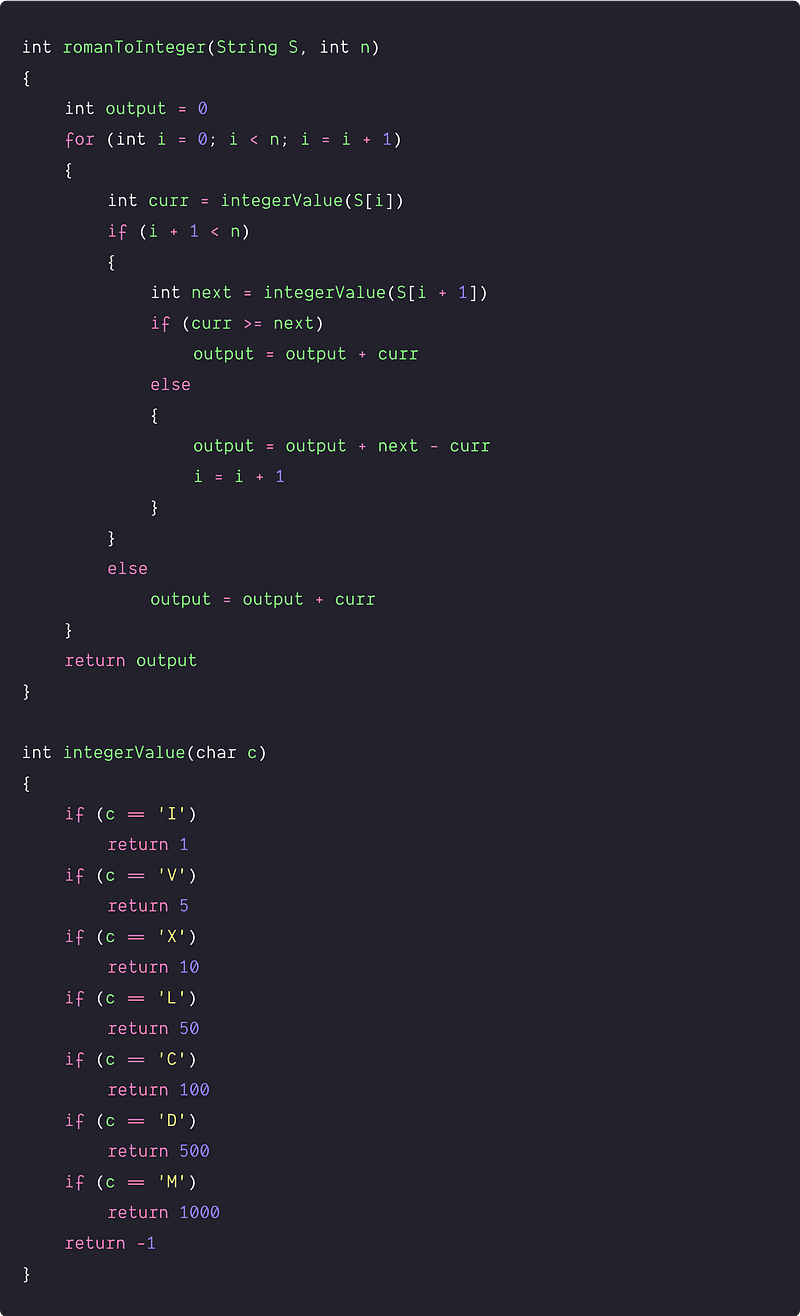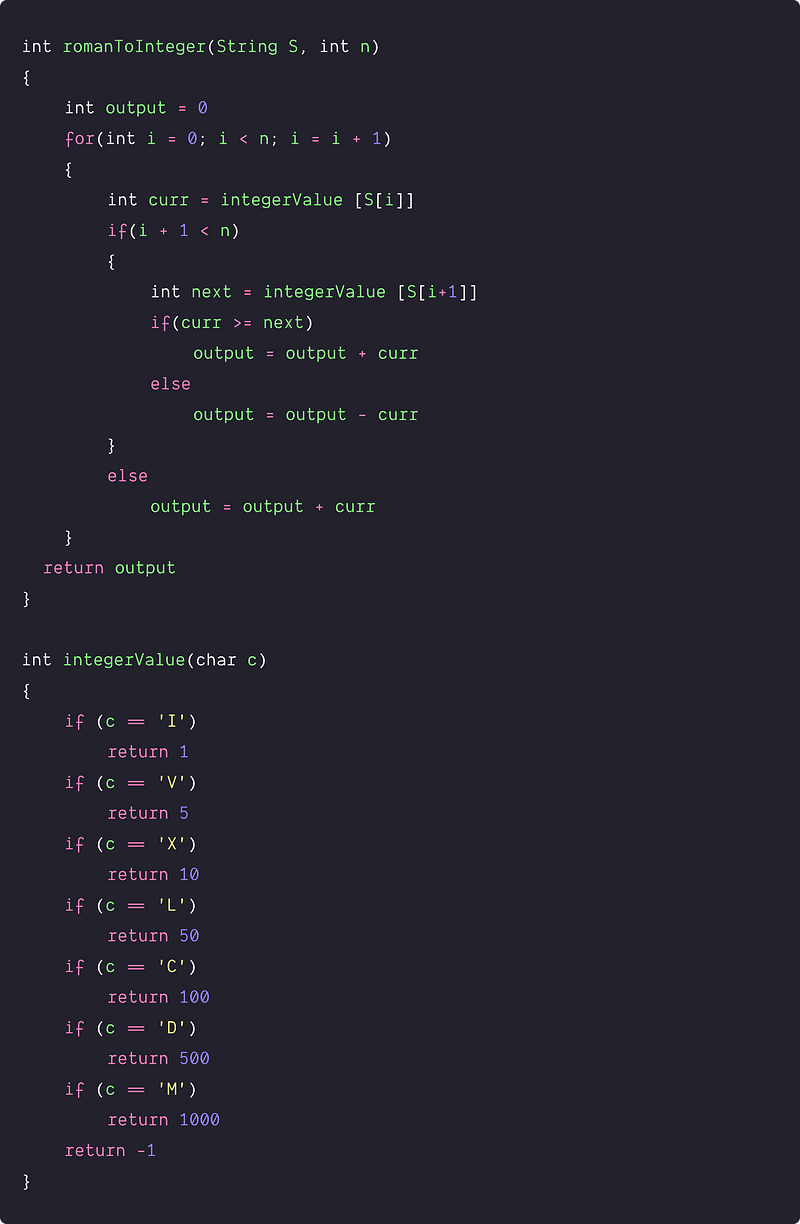# Roman to Integer

Key takeaway: A good mathematical problem to learn problem-solving using loop.

### Let’s understand the problem

Given a Roman numeral, write a program to find its corresponding decimal value. Roman numerals are represented by seven different symbols:

``````Symbol       Value
I             1
V             5
X             10
L             50
C             100
D             500
M             1000
``````

Problem Note

• The input contains only the characters `('I', 'V', 'X', 'L', 'C', 'D', 'M')`.
• Input is a valid roman numeral in the range `[1, 3999]`.
• A number in Roman Numerals is a string of these symbols written in descending order i.e. largest to smallest from left to right. For example, `2` is written as `II` in Roman numeral, just two ones added together. `12` is written as `XII`, which is simply `X + II`. The number `27` is written as `XXVII`, which is `XX + V + II`.
• We avoid four characters being repeated in successions such as IIII or VIIII. Instead, the number four is written as IV because the 1 is before the 5 we subtract it making 4. The same principle applies to number 9, which is written as IX. The idea is : when there is a smaller number placed before a larger number, the values are subtracted.

Example 1

Input: XL, Output: 40

Explanation: XL is a Roman symbol which represents 40

Example 2

Input: MCMIV, Output: 1904

Explanation: M = 1000, CM = 900, IV = 4

Example 3

Input: LVIII, Output: 58

Explanation: L = 50, V= 5, III = 3

Example 4

Input: MCMXCIV, Output: 1994

Explanation: M = 1000, CM = 900, XC = 90 and IV = 4

### Solution using a single scan

Solution Idea and Steps

According to the above properties of the roman numbers, two conditions will appear for any substring of the given input:

• case 1 : The string of symbols will appear in descending order or three characters being repeated in successions.
• case 2 : The smaller roman number is placed before a larger number or four characters is not repeated in the successions.

Now solution idea would be to scan the input string and incrementally calculate the integer value based on the appearance of case 1 and case 2 . Suppose function romanToInteger(String S, int n) is converting a roman string S of size n to its integer value.

• We declare variable output to build the integer value of the given roman string.
• Now we scan the input string using a loop and check each value .
• Inside the loop, we declare two variables curr and next to track the integer value of two consecutive roman characters at index i and i + 1. Here we are using the helper function integerValues(char c) to get the integer value of a roman character.
• If (curr ≥ next): This is a situation of case 1, so we add the curr value to the output i.e. output = output + curr.
• If (curr < next): This is a situation of case 2, so we add (next - curr) to the output i.e. output = output + (next - curr). Here (next - curr) is the combined integer value of the two roman characters, so we also increment the value of i by 1. Note: we are also incrementing i in the loop.
• Boundary condition: before calculating the next, we need to check: are we present at the last index? If yes, then there is no need to calculate next and we just add the curr to the output i.e. output = output + curr.
• By end of the loop, we return the value stored in the variable output.

Solution PseudocodeAnother solution idea similar to the above approach

From the Roman string of a number, we can observe one thing: we can place only one smaller number before a larger number. Or in other words, there will be at max two Roman characters in the increasing order. So the basic idea would be:

• Access each roman character using a loop and check the current character is less than the next character or not. If yes, then we subtract the integer value of the current character from the overall output.
• Otherwise, we add that the integer value of the current character from the overall output. This would be the scenario when string symbols will appear in descending order or three characters being repeated in successions.
• We need to take care of the boundary condition when we reach the last character.Time and space complexity analysis

In both approaches, we are running a single loop and doing O(1) operations at each iteration. So time complexity = n*O(1) = O(n). We are using a constant number of variables, so space complexity = O(1).

### Critical ideas to think!

• Can we solve this problem using some other iterative approach?
• Can we think about solving this problem recursively?
• Instead of using the helper function integerValue(char c), can we implement the above solution using switch statements or an unordered map?
• Which one of the above solutions will perform less number of operations?
• Are there some other boundary conditions missing in the above solutions?

### Suggested problems to practice

• Convert integer to English words
• Convert a decimal number to Roman numerals
• Validate Roman numerals using regular expression
• Find the largest roman numeral possible by rearranging characters of a string

Enjoy learning, Enjoy algorithms, Enjoy problem-solving!

Get well-designed application and interview centirc content on ds-algorithms, machine learning, system design and oops. Content will be delivered weekly.